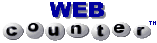# Portfolio Optimization

Robert F. Stambaugh
Miller Anderson & Sherrerd Professor of Finance

To begin the program, choose the number of assets in the portfolio to be analyzed from the following menu. For additional information, read below.

This program performs basic mean-variance optimization with arbitrary bounds on portfolio weights. The user can choose to compute the portfolio that either

• maximizes the mean-variance objective function, or
• improves the value of the function with a two-asset rebalancing.

In the second case, the two assets are chosen in the manner outlined by William Sharpe in

"An Algorithm for Portfolio Improvement,"Advances in Mathematical Programming and Financial Planning, (K.D. Lawrence, J.B. Guerard, Jr., and Gary D. Reeves, Editors), JAI Press, Inc., 1987, pp. 155-170.
and the optimization algorithm, implemented here using MATLAB, also follows Sharpe's approach.

## Inputs

The required inputs consist of

• the coefficient of risk aversion
• expected returns
• standard deviations
• correlations
• initial portfolio weights that sum to 1.0
In addition, an upper and/or lower bound on each of the portfolio weights can be specified. Riskless assets are permitted, and they are indicated simply by entering zero for those assets' standard deviations and correlations with other assets. (Of course, multiple riskless assets are sensible only if appropriate bounds are placed on their weights.)

## Outputs

The program output gives the properties of the initial portfolio as well as the final portfolio. Included are the derivatives of the objective function with respect to each of the weights. In general, for an optimal portfolio, these derivatives should be equal across all weights that are not constrained by a bound.

## Remarks

David Robinson, formerly of Wharton's Finance Department, deserves credit for developing much of the Web interface (Forms, CGI, Perl, etc.). I must take credit for any errors in the optimization program. The program is not bulletproof, although there are a number of checks on inputs, such as whether the correlations are mutually consistent (i.e., whether the covariance matrix is non-negative definite). Some at Wharton may recognize the similarity of the program to the author's earlier implementations of Sharpe's algorithm in a Lotus 123 spreadsheet (SWAP.WK1 or IMPROVE.WK1).

Feedback welcome at stambaugh@wharton.upenn.edu

##### © 1996 Robert F. Stambaugh